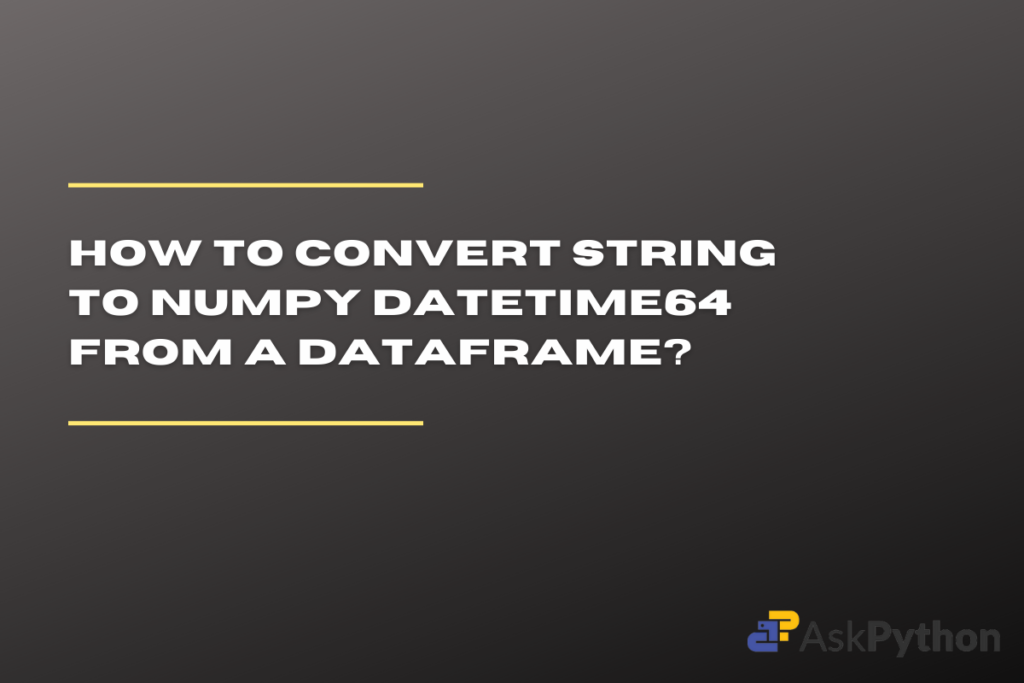# Converting String to Numpy Datetime64 in a DataframePython contains many open-sourced packages to work on datasets. It also supports a diverse range of data types. When it comes to working on data related to date or time, it is preferred to use the datetime data type instead of the string or float data type, as it helps to keep the data uniform. Having a uniform data type with the same format also helps to avoid errors while processing the data.

In this article, let us try to comprehend how to convert data of string data type into Numpy Datetime data type. We will explore multiple approaches using various packages and methods to achieve this objective.

## Different Ways to Convert String to Numpy Datetime64 in a Pandas Dataframe

To turn strings into numpy datetime64, you have three options: Pandas to_datetime(), astype(), or datetime.strptime(). The to_datetime() function is great if you want to convert an entire column of strings. The astype() function helps you change the data type of a single column as well. The strptime() function is better with individual strings instead of dataframe columns.

There are multiple ways you can achieve this result. Here are a few methods to convert a string to numpy datetime64.

### Using Pandas to_datetime() Function

The Pandas package contains many in-built functions which help in modifying the data; one such function is the to_datetime. The primary objective of this function is to convert the provided argument into a datetime format. Before we start with the methodology make sure to install and import the Pandas package in your current working IDE to avoid further errors. Run the following lines of code to do so.

```# To install the package
pip install pandas

# To import the package
import pandas as pd
```

To understand how the function works let us consider a sample dataframe with two columns – date and time. The data type of both columns is ‘object’. By providing the column names to the to_datetime function as the argument, the data type of the columns is converted into datetime. Take a look at the code below for a better understanding.

```df = pd.DataFrame(
{'Date': ["1-10-2000","1-11-2000","1-12-2000"],
'Time': ['1:05:00', '2:10:01', '2:15:02']})
print(df.dtypes)
print("---------")

x = pd.to_datetime(df['Date'])
y = pd.to_datetime(df['Time'])
print("x.dtypes, "\n", y.dtypes)
```

OUTPUT

### Using Pandas astype() Function

The astype() is a simple function provided by the Pandas package. The function is used to convert the data into any other specified data type. The function takes a string argument that specifies the name of the desired data type. The following code will help you to understand how this function is used to convert data from a dataframe into datetime64[ns] data type.

```df = pd.DataFrame(
{'Date': ["1-10-2000","1-11-2000","1-12-2000"],
'Time': ['1:05:00', '2:10:01', '2:15:02']})
print(df.dtypes)
print("---------")

x = df.Date.astype('datetime64[ns]')
y = df.Time.astype('datetime64[ns]')
print(x.dtypes)
print(y.dtypes)
```

OUTPUT

NOTE: The above-discussed method works for variables as well as data frames. As we are discussing on the conversion of string datatype to datetime datatype, the following method is added as a bonus. If you need to change only a particular variable you can use the next method discussed but do remember it doesn’t work for data frames.

### Using strptime() Function from datetime Module

Before we start with the implementation of the method, make sure to import the datetime package and the ‘datetime’ submodule in your working IDE. To do so run the following lines of code in your current IDE.

```import datetime
from datetime import datetime as dt
```

The ‘strptime()’ takes two strings as the input, the first string is the data we need to change into the datetime data type, and the second argument is the format of the date or time which we have provided as the input. This functions in numerous ways of date and time formatting. Click here to learn more about the formatting codes used by the ‘strptime()’ function. As this function considers only string as an argument, this method cant be used for data frames. Take a look at the following code for a better understanding.

```import datetime
from datetime import datetime as dt

x = "10-04-2000"
print(x, type(x))
print("---------")

y = dt.strptime(x, '%d-%m-%Y')
print(y, type(y))
```

OUTPUT